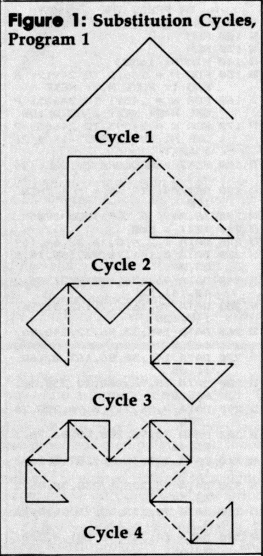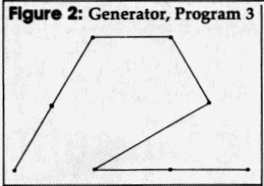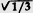`COMPUTE! ISSUE 64 / SEPTEMBER 1985 / PAGE 84`

Apple Fractals

Paul W. Carlson

Fractals are receiving a great deal of attention in mathematics and computergraphics these days. They're being used for everything from simulating random plant growth to generating realistic planetary landscapes for science-fiction films. This article introduces the fascinating world of fractals with three programs that demonstrate a particular type of fractal that can be plotted on a personal computer.

The word fractal was coined by Benoit Mandelbrot, a pioneer in their study, to denote curves or surfaces having fractional dimension. The concept of fractional dimension can be illustrated as follows: A straight curve (a line) is one-dimensional, having only length. However, if the curve is infinitely long and curves about in such a manner as to completely fill an area of the plane containing it, the curve could be considered two-dimensional. A curve partially filling an area would have a fractional dimension between one and two.
Many types of fractals are self-similar, which means that all portions of the fractal resemble each other. Self-similarity occurs whenever the whole is an expansion of some basic building block. In the language of fractals, this basic building block is called the generator. The generator in the accompanying programs consists of a number of connected line segments. The curves that the programs plot are the result of starting with the generator and then repeatedly replacing each line segment with the whole generator according to a defined rule. Theoretically, these replacement cycles would continue indefinitely. In practice, the screen resolution limits the number of cycles.
The programs illustrate two types of fractal curves. The curves generated by Program 1 and Program 2 are self-contacting, while the curve generated by Program 3 is self-avoiding. A self-contacting curve touches itself but does not cross itself. A self-avoiding curve never actually touches itself although it may appear to because of the limited screen resolution.

The Dragon Sweep
Program 1 plots what Mandelbrot refers to as a "dragon sweep." It demonstrates in a step-by-step fashion how a fractal curve is filled. The generator consists of two-line segments of equal length forming a right angle. During each replacement cycle, the generator is substituted for each segment on alternating sides of the segments, that is, to the left of the first segment, to the right of the second segment, and so on. Figure 1 shows the first few cycles of substitution. The program is written in BASIC so the plotting is slow enough to let you observe the development of the curve.
The program prompts you to enter an even number of cycles (for reasons of efficiency and screen resolution, only even numbers of cycles are plotted). When a plot is complete, pressing any key clears the screen and returns you to the prompt. I recommend starting with two cycles, then four, six, etc. It takes fourteen cycles to completely fill in the "dragon," but since this requires almost two hours, you will probably want to quit after about ten cycles. You can see the complete dragon by running Program 2, which always plots the dragon first in less than 30 seconds.
Since it's not at all obvious how the program works, here's a brief explanation. NC is the number of cycles; C is the cycle number; SN is an array of segment numbers indexed by cycle number; L is the segment length; D is the segment direction, numbered clockwise from the positive x direction; and X and Y are the high-resolution screen coordinates.

 Lines 100-140 Get number of cycles from user. Line   150 Computes segment length. Line   160 Sets starting coordinates. Line   170 Sets segment numbers for all cycles to the first segment. Lines 180-220 Find the direction of the segment in the last cycle by rotating the segment in each cycle that will contain the segment in the last cycle. Lines 230-260 Increase or decrease X or Y by the segment length, depending on the seg ment direction. Lines 270-290 Plot the segment and update the current segment number for each cycle. Lines 300-320 If the segment number for cycle zero is still zero, do the next segment; otherwise, we're done.

Eight Thousand Dragons
Program 2 plots more than 8,000 different dragons. It does this by randomly determining on which side of the first segment the generator will be substituted for all cycles after the first cycle. The generator is always substituted to the left of the first segment in the first cycle to avoid plotting off the screen. Other than the randomization, this program uses the same logic as Program 1. The main part of this program is written in machine language to reduce the time required to plot a completely filled-in dragon from about two hours to less than half a minute.
All the dragons are plotted after fourteen cycles of substitution. All have exactly the same area, which equals half of the square of the distance between the first and last points plotted. All the dragons begin and end at the same points.
When a plot is complete, press the space bar to plot another dragon, or press the Q key to quit.

Snowflakes
Program 3 plots what Mandelbrot refers to as a "snowflake sweep." The generator, shown in Figure 2, was discovered by Mandelbrot. The segments are numbered zero through six, starting at the right. The program is basically the same as Program 1. The variables NC, C, SN, D, X, and Y represent the same values except that the direction D is numbered counterclockwise from the negative x direction. For each segment, the accompanying table gives the value of RD (relative direction), LN (length factor), and SD (flags indicating which side of the segment the generator is to be placed).

 Line   20 Reads values of SD and RD. Compute LN values. Lines 30-50 Compute delta x and delta y factors for each direction. Lines 60-100 Get number of cycles from user. Line   120 Sets starting coordinates. Line   130 Sets the segment numbers for all cycles to the first segment. Lines 140-170 Find the direction of the segment in the last cycle. Lines 180-190 Compute the coordinates of the end of the segment, plot the segment, and update the segment numbers for each cycle. Lines 200-220 Same as lines 300-320 in Program 1.

Like Program 1, pressing any key when a plot is complete clears the screen and brings another prompt.Experlment!
I hope these programs encourage you to look further into the fascinating world of fractals. Don't be afraid to experiment with the programstry modifying the shape of the generator in Program 3, for example. Better yet, design your own generator.
These programs just begin to explore the possibilities of fractal computer graphics. There is another whole class of fractals, those generated by functions of complex variables. And then there are three-dimensional fractals. And then....Values For Program 3 Segment Number SN Relative Direction RD Length Factor LN Side  Flag SD 0 0 1/3 0 1 0 1/3 1 2 71 3 10 1/3 0 4 0 1/3 0 5 2 1/3 0 6 2 1/3 1

Program 1: The Dragon Sweep

1E 10 REM PROGRAM 1
6A 20 REM
78 30 REM THIS PROGRAM PLOTS A
FRACTAL "DRAGON SWEEP"
D0 40 REM FOR AN EVEN NUMBER OF
CYCLES (14 MAX).
60 50 REM
9D 90 DIM SN(14)
54 100 TEXT : HOME
F1 110 PRINT "ENTER AN EVEN NO.
OF CYCLES (2 TO 14)"
90 120 INPUT "          OR ENTER
A ZERO TO QUIT: ";NC
A7 130 IF NC = 0 THEN END
E4 140 IF INT (NC / 2) * 2 < > N
C OR NC < 2 OR NC > 14 TH
EN 100
1D 150 L = 128: FOR C = 2 TO NC
STEP 2:L = L / 2: NEXT
E8 160 X = 77:Y = 128: HGR2 : HC
OLOR= 3: HPLOT X,Y
81 170 FOR C = 0 TO NC:SN(C) = 0
: NEXT
43 180 D = 0: FOR C = 1 TO NC: I
F SN(C - 1) = SN(C) THEN
D = D - 1: GOTO 200
46 190 D=D+ 1
ED 200 IF D = - 1 THEN D = 7
1C 210 IF D = 8 THEN D = 0
FD 220 NEXT
90 230 IF D = 0 THEN X = X + L:
GOTO 270
F0 240 IF D = 2 THEN Y = Y + L:
GOTO 270
A4 250 IF D = 4 THEN X = X - L:
GOTO 270
9A 260 Y = Y - L
35 270 HPLOT TO X,Y:SN(NC) = SN(
NC) + 1
10 280 FOR C = NC TO 1 STEP - 1:
IF SN(C) < > 2 THEN 300
9F 290 SN(C) = 0:SN(C - 1) - SN(
C - 1) + 1: NEXT
BA 300 IF SN(0) = 0 THEN 180
D6 310 GET A\$: IF A\$ = "" THEN 3
10
90 320 GOTO 100

Program 2: Eight Thousand Dragons

2E 10 REM PROGRAM 2
6A 20 REM
6B 30 REM
92 40 REM THIS PROGRAM PLOTS RA
NDOM FRACTAL "DRAGON SWEEP
S."
7C 50 REM THE "STANDARD" DRAGON
IS ALWAYS PLOTTED FIRST.
6E 60 REM
5F 70 REM WHEN A PLOT IS COMPLE
TE, PRESS THE SPACE BAR
D1 80 REM TO PLOT ANOTHER DRAGO
N, OR PRESS THE "Q" KEY
97 90 REM TO EXIT THE PROGRAM.
82 100 REM
86 130 REM
6B 140 HIMEM: 16383
DO 150 FOR N = 24612 TO 24912: R
9F 160 FOR N = 24591 TO 24605: P
OKE N,0: NEXT : GOTO 180
17 170 FOR N = 24593 TO 24605: P
OKE N, INT ( RND (1) * 2)
: NEXT
24 180 HGR2 : HCOLOR= 3: CALL 24
619
85 190 GET AS: IF A\$ = " " THEN
170
D8 200 IF A\$ < > "Q" THEN 190
FF 210 TEXT : END
F6 220 DATA 1,2,4,8,16,32,64,169
64 230 DATA 0,141,16,96,160,14,1
53,0
1C 240 DATA 96,136,192,255,208,2
48,141,32
AF 250 DATA 96,162,77,142,31,96,
160,128
22 260 DATA 140,33,96,32,248,96,
169,0
A5 270 DATA 141,30,96,162,0,160,
1,185
DB 280 DATA 15,96,208,20,238,30,
96,189
26 290 DATA 0,96,217,0,96,208,26
,206
2B 300 DATA 30,96,206,30,96,76,1
25,96
AB 310 DATA 206,30,96,189,0,96,2
17,0
26 320 DATA 96,208,6,238,30,96,2
38,30
85 330 DATA 96,173,30,96,16,5,16
9,7
AF 340 DATA 141,30,96,201,8,208,
5,169
16 350 DATA 0,141,30,96,232,200,
224,14
DO 360 DATA 208,189,170,208,20,1
73,31,96
17 370 DATA 24,105,1,141,31,96,1
73,32
4D 380 DATA 96,105,0,141,32,96,7
6,210
7A 390 DATA 96,224,2,208,6,238,3
3,96
44 400 DATA 76,210,96,224,4,208,
20,173
0C 410 DATA 31,96,56,233,1,141,3
1,96
53 420 DATA 173,32,96,233,0,141,
32,96
E1 430 DATA 76,210,96,206,33,96,
32,248
15 440 DATA 96,238,14,96,160,14,
162,13
68 450 DATA 185,0,96,201,2,208,1
2,169
84 460 DATA 0,153,0,96,254,0,96,
202
CF 470 DATA 136,208,237,173,0,96
,208,3
E1 480 DATA 76,74,96,96,173,33,9
6,10
D1 490 DATA 10,41,28,9,64,133,27
,173
28 500 DATA 33,96,74,74,74,74,41
,3
FF 510 DATA 5,27,133,27,173,33,9
6,41
45 520 DATA 192,72,106,133,26,10
4,74,74
1F 530 DATA 74,5,26,133,26,173,3
1,96
8F 540 DATA 141,34,96,173,32,96,
141,35
66 550 DATA 96,56,160,255,200,17
3,34,96
fC 560 DATA 233,7,141,34,96,173,
35,96
35 570 DATA 233,0,141,35,96,16,2
37,173
FC 580 DATA 34,96,105,7,170,189,
36,96
71 590 DATA 17,26,145,26,96

Program 3: The Snowflake Sweep

3E 10 REM PROGRAM 3
6A 20 REM
B1 30 REM THIS PROGRAM PLOTS A
FRACTAL "SNOWFLAKE SWEEP"
6C 40 REM
9C 50 DIM DX(11),DY(11):M = 7 /
6
1C 60 FOR N = 0 TO 6: READ SD(N)
,RD(N):LN(N) = 1 / 3: NEXT
:LN(2) = SQR (LN(1))
F1 70 A = 0:    FOR D = 6 TO    11: DX
D) = COS (A):DY(D) = SIN
A)
BC 80 A = A + 0.52359879: NEXT
EB 90 FOR D = 0 TO 5:DX(D) = - D
X(D + 6):DY(D) = - DY(D +
6): NEXT
54 100 TEXT : HOME
85 110 PRINT "ENTER NUMBER OF CY
CLES ( 1 - 4 )"
90 120 INPUT "         OR ENTER
A ZERO TO QUITS ";NC
A7 130 IF NC = 0 THEN END
1A 140 IF NC > 4 THEN 100
9D 150 HGR2 : HCOLOR= 3
BE 160 X = 235:Y = 142:TL = 162:
HPLOT X,Y
81 170 FOR C = 0 TO NC:SN(C) = 0
: NEXT
04 180 D = 0:L = TL:NS = 0: FOR
C = 1 TO NC:I = SN(C):L =
L * LN(I):J = SN(C - 1):
NS = NS + SD(J):K = INT (
NS / 2): IF K * 2 < > NS
THEN D = D + 12 - RD(I):
GOTO 200
61 190 D = D + RD(I)
92 200 IF D > 11 THEN D = D - 12
FB 210 NEXT
70 220 X = X + M * L * DX(D):Y =
Y - L * DY(D): HPLOT TO
X,Y:SN(NC) = SN(NC) + 1:
FOR C = NC TO 1 STEP - 1:
IF SN(C) < > 7 THEN 240
93 230 SN(C) = 0:SN(C - 1) = SN(
C - 1) + l: NEXT
C1 240 IF SN(0) = 0 THEN 180
4E 250 GET A\$: IF A\$ = "" THEN 2
50
97 260 GOTO 100
41 270 DATA 0,0,1,0,1,7,0,10,0,0
,0,2,1,2